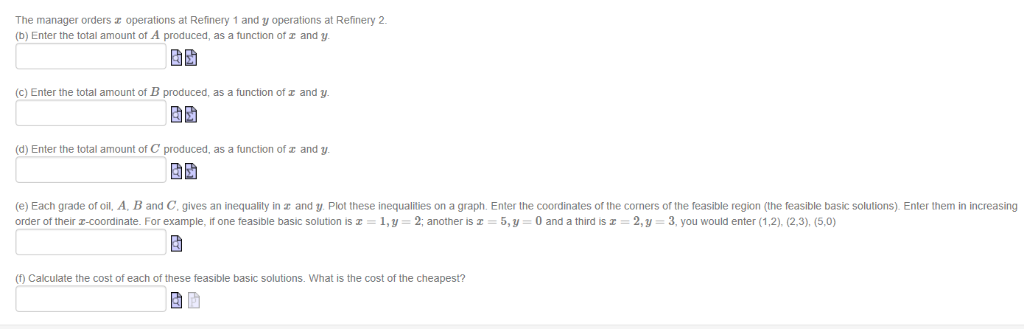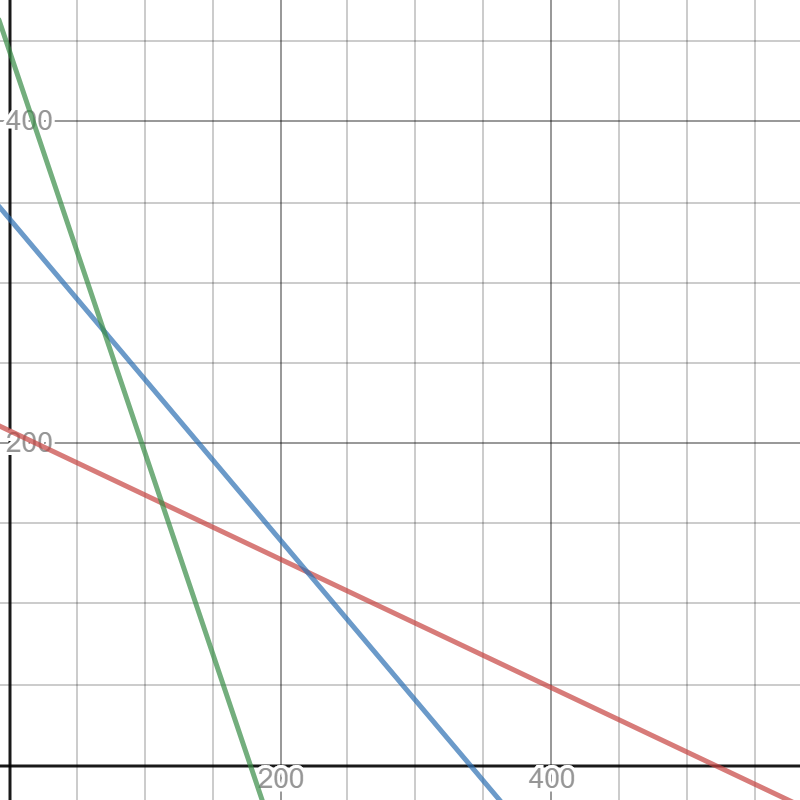# Two oil refineries each produce three grades of petroleum, A, B and C. At each refinery, the various grades One operati...Two oil refineries each produce three grades of petroleum, A, B and C. At each refinery, the various grades One operation at Refinery 1 produces 2 units of A, 1 unit of B and 5 units of C f petroleum are produced in a single operation so that they are fixed proportions. One operation at Refinery 2 produces 5 units of A, 1 unit of B and 2 units of C. Refinery 1 charges \$200 for one operation, and Refinery 2 charges \$350 for one operation. consumer needs 1,040 units of A, 340 units of B and 890 units of C How should the orders be placed ir the consumer's needs are to be met most economically?
The manager orders operations at Refinery 1 and y operations at Refinery 2 (b) Enter the total amount of a function of r and y produced, (c) Enter the total amount of B produced, as a function of and y produced, a function of (d) Enter the total amount of and y (e) Each grade of oil. A. B and C. gives an inequality inz and y. Plot these inequalities on a graph, Enter the coordinates of the comers of the feasible region (the feasible basic solutions), Enter them i increasing order of their T-COordinate. For example, if one feasible basic solution is = 1,y 2, another is = 5, y 0 and a third is 2,y 3, you would enter (1,2), (2,3), (5,0) f these feasible basic solutions. What is the cost of the cheapest? (f) Calculate the cost of each

Let there be x operations at Refinery 1 and y operations at the Refinery 2.

(b). The total amount of A produced is 2x+5y.

( c). The total amount of B produced is x+y.

(d). The total amount of C produced is 5x+2y

(e). In view of the requirements of the consumer, we have 2x+5y ≥ 1040…(1), x+y ≥ 340…(2) and 5x+2y ≥ 890…(3).

A graph of the lines 2x+5y = 1040 (in red), x+y = 340 (in blue) and 5x+2y = 890 (in green) is attached. The feasible region is on and above all these 3 lines.

The blue and the green lines intersect at A: ( 70,270) and the blue and the red lines intersect at B: (220,120). Thus, the only feasible basic solutions are (70,270) and (220,120).

(f). At A, the cost of operation of the 2 refineries is 70*200+270*350 = 14000+94500 = \$ 108500.

At B, the cost of operation of the 2 refineries is 220*200+120*350 = 44000+42000 = \$ 86000.

The cheapest cost is \$ 86000 from 220 operations at Refinery 1 and 120 operations at the Refinery 2.-400- 200 200 400

##### Add Answer of: Two oil refineries each produce three grades of petroleum, A, B and C. At each refinery, the various grades One operati...
More Homework Help Questions Additional questions in this topic.